## Example Questions

### Example Question #26 : Trigonometry

What is the tangent of the angle formed between the origin and the point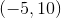if that angle is formed with one side of the angle beginning on the-axis and then rotating counter-clockwise to?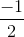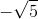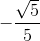Explanation:

You can begin by imagining a little triangle in the second quadrant to find your reference angle. It would look like this: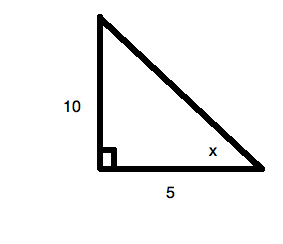The tangent of an angle is: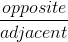For our data, this is: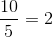Now, since this is in the second quadrant, the value is negative, given the periodic nature of the tangent function.

### Example Question #27 : Trigonometry

What is the tangent of the angle formed between the origin and the point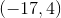if that angle is formed with one side of the angle beginning on the-axis and then rotating counter-clockwise to? Round to the nearest hundredth.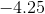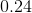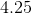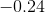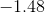Explanation:

Recall that when you calculate a trigonometric function for an obtuse angle like this, you always use the-axis as your reference point for your angle. (Hence, it is called the "reference angle.")

Now, it is easiest to think of this like you are drawing a little triangle in the second quadrant of the Cartesian plane. It would look like: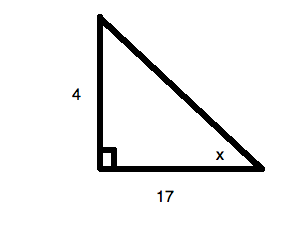So, the tangent of an angle is:or, for your data,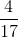.

This is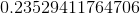. Rounding, this is. However, sinceis in the second quadrant, your value must be negative. (The tangent function is negative in that quadrant.) Therefore, the answer is.

### Example Question #28 : Trigonometry

What is the tangent of the angle formed between the origin and the point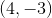if that angle is formed with one side of the angle beginning on the-axis and then rotating counter-clockwise to? Round to the nearest hundredth.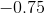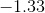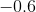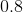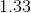Explanation:

Recall that when you calculate a trigonometric function for an obtuse angle like this, you always use the-axis as your reference point for your angle. (Hence, it is called the "reference angle.")

Now, it is easiest to think of this like you are drawing a little triangle in the fourth quadrant of the Cartesian plane. It would look like: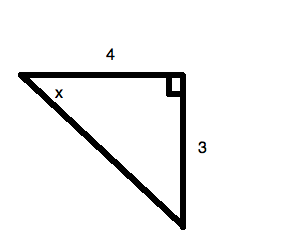So, the tangent of an angle is:or, for your data,or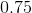. However, sinceis in the fourth quadrant, your value must be negative. (The tangent function is negative in that quadrant.) This makes the correct answer.